# 基于HUST-Ship的方波数值造波方法研究 Numerical Studies of Square Wave Generation Using HUST-Ship

DOI: 10.12677/AMS.2020.74016, PDF, HTML, XML, 下载: 113  浏览: 484

Abstract: When two waves in different directions meet due to different weather patterns, a cross wave pattern will be generated, which will finally result in the spread of square waves on the sea surface. The emergence of square waves will threaten the navigation performance of the ship and may lead to ship capsized in severe case. In this paper, the numerical square wave flume is developed by using the in-house CFD software HUST-Ship. In the process of data simulation, by means of solving the RANS equation of incompressible fluid, the analytical solution of the corresponding regular wave is imposed at the inlet boundary, and the free surface is captured by level-set method. Two regular waves with a cross angle of 90 degrees and the same wavelength and phase are superimposed. The probe is used to deal with the simulation results and trace the selected points to obtain the relative errors between the simulation results and the analytical solutions. Moreover, error analysis is used to ensure the accuracy of the numerical tank.

1. 引言

2. 流体控制方程和数值方法

2.1. 控制方程

HUST-Ship采用有限差分法对URANS方程进行离散，采用水平集法对自由液面进行捕获。不可压缩连续方程和RANS方程如下：

$\frac{\partial {u}_{i}}{\partial {x}_{i}}=0$ (1)

$\frac{\partial \stackrel{¯}{{u}_{i}{u}_{j}}}{\partial {x}_{j}}=-\frac{1}{\rho }\frac{\partial p}{\partial {x}_{i}}+\frac{\partial }{\partial {x}_{j}}\left[\gamma \left(\frac{\partial \stackrel{¯}{{u}_{i}}}{\partial {x}_{j}}+\frac{\partial \stackrel{¯}{{u}_{j}}}{\partial {x}_{i}}\right)\right]-\frac{\partial \left(-\stackrel{¯}{{{u}^{\prime }}_{i}{{u}^{\prime }}_{j}}\right)}{\partial {x}_{j}}+{f}_{i}$ (2)

$\frac{\partial \phi }{\partial t}+v\nabla \phi =0$ (3)

$\nabla v\cdot {n}_{j}=0,\text{\hspace{0.17em}}\text{\hspace{0.17em}}{n}_{j}=\frac{\partial \phi /\partial {x}_{i}}{|\partial \phi /\partial {x}_{i}|}$ (4)

2.2. 造波与消波的方法

$\eta \left(t\right)=A\mathrm{cos}\left(kx-\omega t+\phi \right)$ (5)

$u=A\omega \frac{\mathrm{cosh}k\left(z+d\right)}{\mathrm{sinh}kd}\mathrm{sin}\left(kx-\omega t+\phi \right)$ (6)

$w=A\omega \frac{\mathrm{sinh}k\left(z+d\right)}{\mathrm{sinh}kd}\mathrm{sin}\left(kx-\omega t+\phi \right)$ (7)

$\frac{\partial u}{\partial t}+u\frac{\partial u}{\partial x}+v\frac{\partial u}{\partial y}={g}_{x}-\frac{1}{\rho }\frac{\partial p}{\partial x}+v\left[\frac{{\partial }^{2}u}{\partial {x}^{2}}+\frac{{\partial }^{2}u}{\partial {y}^{2}}\right]-\mu \left(x\right)u$ (8)

$\frac{\partial v}{\partial t}+u\frac{\partial v}{\partial x}+v\frac{\partial v}{\partial y}={g}_{y}-\frac{1}{\rho }\frac{\partial p}{\partial y}+v\left[\frac{{\partial }^{2}v}{\partial {x}^{2}}+\frac{{\partial }^{2}v}{\partial {y}^{2}}\right]-\mu \left(x\right)v$ (9)

$\mu \left(x\right)=\left\{\begin{array}{l}{a}_{s}\left(x-{x}_{0}\right)/{L}_{s}\text{\hspace{0.17em}}\text{\hspace{0.17em}}\text{\hspace{0.17em}}\text{\hspace{0.17em}}x>{x}_{0}\\ 0\text{\hspace{0.17em}}\text{\hspace{0.17em}}\text{\hspace{0.17em}}\text{\hspace{0.17em}}\text{\hspace{0.17em}}\text{\hspace{0.17em}}\text{\hspace{0.17em}}\text{\hspace{0.17em}}\text{\hspace{0.17em}}\text{\hspace{0.17em}}\text{\hspace{0.17em}}\text{\hspace{0.17em}}\text{\hspace{0.17em}}\text{\hspace{0.17em}}\text{\hspace{0.17em}}\text{\hspace{0.17em}}\text{\hspace{0.17em}}\text{\hspace{0.17em}}\text{\hspace{0.17em}}\text{\hspace{0.17em}}\text{\hspace{0.17em}}x\le {x}_{0}\end{array}$ (10)

${x}_{0}$ 为消波开始起作用的位置； ${L}_{s}$ 为阻尼层总长度； ${a}_{s}$ 为消波的强度。消波强度是一个经验值。值越大，消波强度越大。但是，如果这个值太大，可能会产生负面影响，导致更严重的反射波。因此，有必要选择一个合理的消波强度值，以获得更好的模拟结果。

3. 数值波浪水池

4. 方波数值造波和误差分析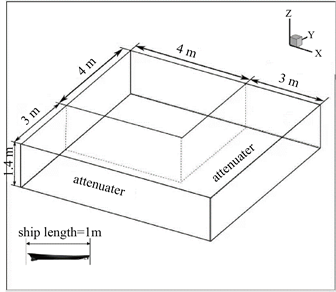Figure 1. Dimension of the computation domain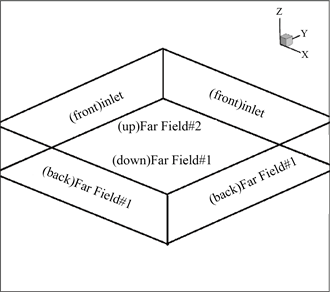Figure 2. Boundary condition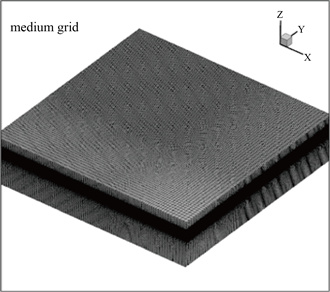Figure 3. Grid distribution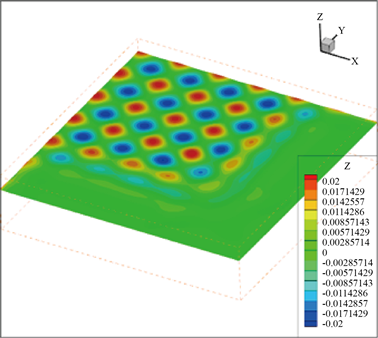Figure 4. Numerical results of square wave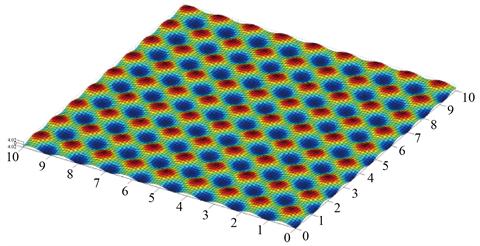Figure 5. Analytical results of square wave

$x=0.02\mathrm{cos}\left(5.461x-13.884t\right)+0.02\left(-5.461y-13.884t\right)$ (11)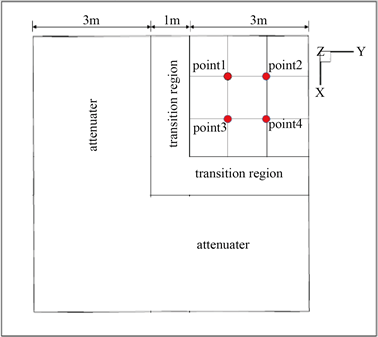Figure 6. Monitor points of wave amplitudes in computational domain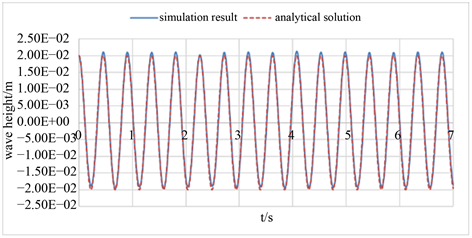Figure 7. Comparison of wave amplitude between numerical and analytical results at point 1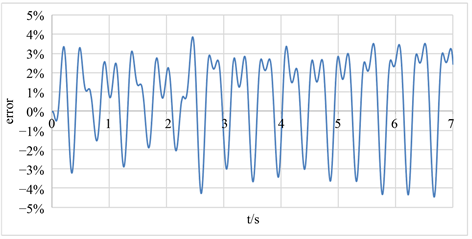Figure 8. Comparison of wave amplitude difference between numerical and analytical results at point 1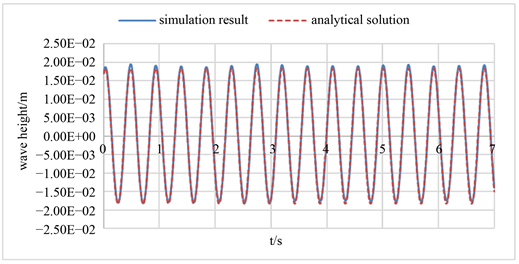Figure 9. Comparison of wave amplitude between numerical and analytical results at point 2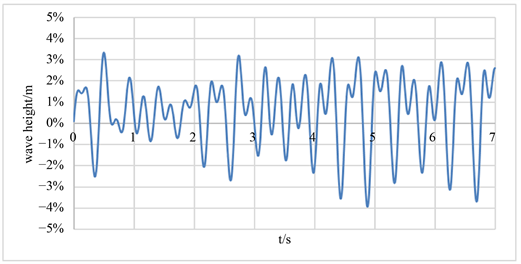Figure 10. Comparison of wave amplitude difference between numerical and analytical results at point 2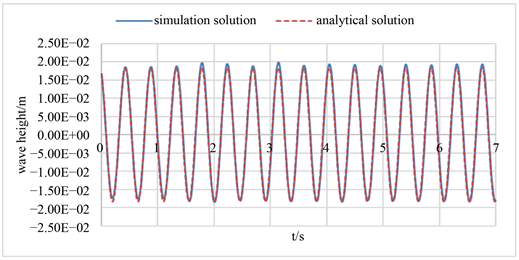Figure 11. Comparison of wave amplitude between numerical and analytical results at point 3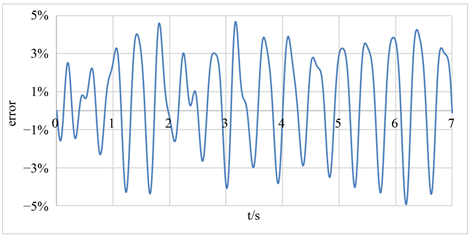Figure 12. Comparison of wave amplitude difference between numerical and analytical results at point 3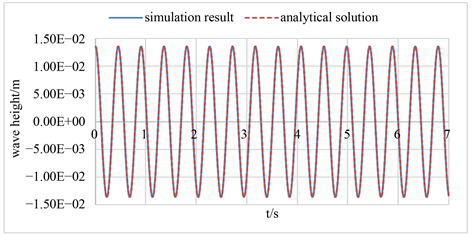Figure 13. Comparison of wave amplitude between numerical and analytical results at point 4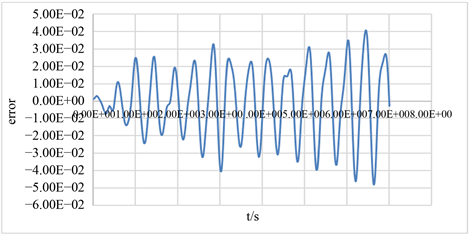Figure 14. Comparison of wave amplitude difference between numerical and analytical results at point 4

5. 结论

  Janssen, P (2003) Nonlinear Four-Wave Interactions and Freak Waves. Journal of Physical Oceanography, 33, 863-884. https://doi.org/10.1175/1520-0485(2003)33<863:NFIAFW>2.0.CO;2  Onorato, M., Osborne, A.R. and Serio, M. (2006) Modulational Instability in Crossing Sea States: A Possible Mechanism for the Formation of Freak Waves. Physical Review Letters, 96, Article ID: 0145031. https://doi.org/10.1103/PhysRevLett.96.014503  Toffoli, A., Bitner-Gregersen, E.M,. Osborne, A.R., Serio, M., Monbaliu, J, and Onorato, M. (2011) Extreme Waves in Random Crossing Seas: Laboratory Experiments and Nu-merical Simulations. Geophysical Research Letters, 38, No. L06605. https://doi.org/10.1029/2011GL046827  Cavaleri, L., Bertotti, L., Torrisi, L., Bitner-Gregersen, E., Serio, M. and Onorato, M. (2012) Rogue Waves in Crossing Seas: The Louis Majesty Accident. Journal of Geophysical Re-search-Oceans, 117, No. C00J10. https://doi.org/10.1029/2012JC007923  Toffoli, A., et al. (2011) Extreme Waves in Random Crossing Seas: Laboratory Experiments and Numerical Simulations. Geophysical Research Letters, 38, No. L06605.  Senthilkumar, R., Romolo, A., Fiamma, V., Arena, F. and Murali, K. (2015) Analysis of Wave Groups in Crossing Seas Using Hilbert Huang Transformation. In: Sundar, V., Sannasiraj, S.A., Murali, K. and Sriram, V., Eds., Procedia Engineering, Elsevier Science BV, Amsterdam, 1042-1049. https://doi.org/10.1016/j.proeng.2015.08.341  Gramstad, O., Bit-ner-Gregersen, E., Trulsen, K. and Nieto Borge, J.C. (2018) Modulational Instability and Rogue Waves in Crossing Sea States. Journal of Physical Oceanography, 48, 1317-1331. https://doi.org/10.1175/JPO-D-18-0006.1  Brennan, J., Dudley, J.M. and Dias, F. (2018) Extreme Waves in Crossing Sea States. International Journal of Ocean and Coastal Engineering, 1, Article ID: 1850001. https://doi.org/10.1142/S252980701850001X  Carrica, P.M., Wilson, R.V. and Stern, F. (2007) An Unsteady Single-Phase Level Set Method for Viscous Free Surface Flows. International Journal for Numerical Methods in Fluids, 53, 229-256. https://doi.org/10.1002/fld.1279  Zhao, F., Chen, J. and Gao, C. (2008) Difference RANS Simu-lation with Free Surface. The 21st National Symposium on Hydrodynamics and the 8th National Academic Conference on Hydrodynamics and the Cross-Strait Symposium on Hydrodynamics of Ships and Marine Engineering, Jinan, 2008, 8.  Lin, P.Z. and Liu, P. (1998) A Numerical Study of Breaking Waves in the Surf Zone. Journal of Fluid Mechan-ics, 359, 239-264. https://doi.org/10.1017/S002211209700846X  Baudic, S.F., Williams, A.N. and Kareem, A. (2001) A Two-Dimensional Numerical Wave Flume—Part 1: Nonlinear Wave Generation, Propagation, and Absorption. Journal of Offshore Mechanics and Arctic Engineering-Transactions of the ASME, 123, 70-75. https://doi.org/10.1115/1.1365117